# 659 Jack O’lantern Puzzle

659 is the sum of the 7 prime numbers from 79 to 107. Can you list them all before you add them up?

Enjoy this Jack O’lantern puzzle.Print the puzzles or type the solution on this excel file: 10 Factors 2015-10-26

———————————————

• 659 is a prime number.
• Prime factorization: 659 is prime.
• The exponent of prime number 659 is 1. Adding 1 to that exponent we get (1 + 1) = 2. Therefore 659 has exactly 2 factors.
• Factors of 659: 1, 659
• Factor pairs: 659 = 1 x 659
• 659 has no square factors that allow its square root to be simplified. √659 ≈ 25.670995.How do we know that 659 is a prime number? If 659 were not a prime number, then it would be divisible by at least one prime number less than or equal to √659 ≈ 25.7. Since 659 cannot be divided evenly by 2, 3, 5, 7, 11, 13, 17, 19, or 23, we know that 659 is a prime number.

———————————

Since riccardo took the time to photograph the puzzle’s solution, I decided to include it in this post as well. Great job riccardo!

# 653 and Level 4

When the last two digits of 653 are divided by 4, we get a remainder of 1 so there is an alternate way to tell if 653 is a prime number by first determining if it is the sum of any two perfect squares:

653 – 1 – 3 – 5 – 7 – 9 – 11 – 13 – 15 – 17 – 19 – 21 – 23 – 25 = 484 which is 22².

653 – 22² = 169 which is 13².

Thus 22² + 13² = 653, and 653 is the sum of two perfect squares.

From that mathematical statement we observe the following:

• 22 and 13 have no common prime factors, and 653 is odd.
• 653 is the hypotenuse of the primitive Pythagorean triple 315-572-653 which was calculated using 22² – 13², 2(22)(13), 22² + 13².
• The only primitive Pythagorean triple hypotenuses less than √653 are 5, 13, and 17.
• 653 obviously is not divisible by 5 or 13.
• If 653 is not divisible by 17, it is a prime number. 653 is not divisible by 17.

Print the puzzles or type the solution on this excel file: 12 Factors 2015-10-19

——————————————————————

• 653 is a prime number.
• Prime factorization: 653 is prime.
• The exponent of prime number 653 is 1. Adding 1 to that exponent we get (1 + 1) = 2. Therefore 653 has exactly 2 factors.
• Factors of 653: 1, 653
• Factor pairs: 653 = 1 x 653
• 653 has no square factors that allow its square root to be simplified. √653 ≈ 25.55386.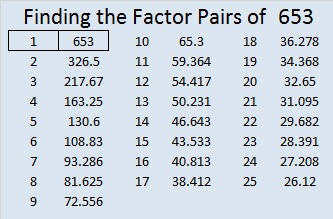How do we know that 653 is a prime number? If 653 were not a prime number, then it would be divisible by at least one prime number less than or equal to √653 ≈ 25.6. Since 653 cannot be divided evenly by 2, 3, 5, 7, 11, 13, 17, 19, or 23, we know that 653 is a prime number.

We also know that 653 is a prime number from the observations made at the beginning of this post.

—————————————————————————————————# 631 and Level 3

631 is a prime number that happens to also be the sum of the eleven prime numbers from 37 to 79.

Sometimes mathematicians talk about triangular numbers, centered triangular numbers, hexagonal numbers, and centered hexagonal numbers. Is there any relationship between those terms?

• The sum of any three consecutive triangular numbers is a centered triangular number.
• ALL hexagonal numbers are triangular numbers.
• Most centered hexagonal numbers are NOT centered triangular numbers.

In my last post, I pointed out that 630 is a triangular number and a hexagonal number.

Well, amazingly the next number, 631, is a centered triangular number AND a centered hexagonal number.

How often does one more than a triangular number equal a centered triangular number? Also how often does one more than a hexagonal number equal a centered hexagonal number?

Let’s compare a couple of lists of these types of numbers up to 631. Red means a number is in both lists. Blue means the number in the 1st list is one less than the number in the 2nd list.

• Triangular numbers (with hexagonal numbers in bold type):
• 1, 3, 6, 10, 15, 21, 28, 36, 45, 55, 66, 78, 91, 105, 120, 136, 153, 171, 190, 210, 231, 253, 276, 300, 325, 351, 378, 406, 435, 465, 496, 528, 561, 595, 630.
• Centered triangular numbers (with numbers that are also centered hexagonal numbers in bold type):
• 1, 4, 10, 19, 31, 46, 64, 85, 109, 136, 166, 199, 235, 274, 316, 361, 409, 460, 514, 571, 631.

1 is the only number in the lists that is a triangular, centered triangular, hexagonal, and centered hexagonal number all at the same time. (1 is also a square number, a pentagonal number, etc.)

(630, 631) is the first combination of numbers that has BOTH a triangular number immediately followed by a centered triangular number AND a hexagonal number immediately followed by a centered hexagonal number.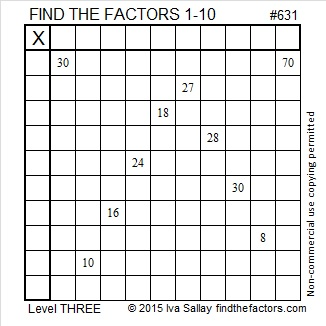Print the puzzles or type the solution on this excel file: 10 Factors 2015-09-28

—————————————————————————————————

• 631 is a prime number.
• Prime factorization: 631 is prime.
• The exponent of prime number 631 is 1. Adding 1 to that exponent we get (1 + 1) = 2. Therefore 631 has exactly 2 factors.
• Factors of 631: 1, 631
• Factor pairs: 631 = 1 x 631
• 631 has no square factors that allow its square root to be simplified. √631 ≈ 25.11971.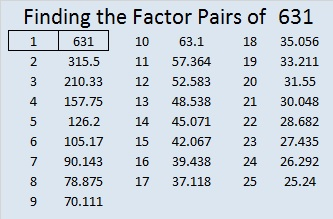How do we know that 631 is a prime number? If 631 were not a prime number, then it would be divisible by at least one prime number less than or equal to √631 ≈ 25.1. Since 631 cannot be divided evenly by 2, 3, 5, 7, 11, 13, 17, 19, or 23, we know that 631 is a prime number.

—————————————————————————————————

A Logical Approach to solve a FIND THE FACTORS puzzle: Find the column or row with two clues and find their common factor. (None of the factors are greater than 10.)  Write the corresponding factors in the factor column (1st column) and factor row (top row).  Because this is a level three puzzle, you have now written a factor at the top of the factor column. Continue to work from the top of the factor column to the bottom, finding factors and filling in the factor column and the factor row one cell at a time as you go.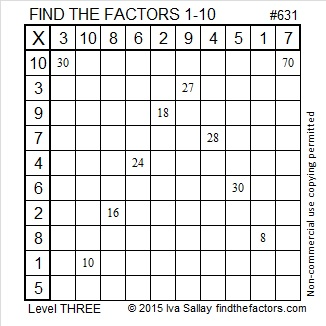# 617 Do You See a Pattern?

If you divide any even square number by 4, there will be no remainder.

If you divide any odd square number by 4, the remainder is always 1.

So if you add an even square number to an odd square number and divide the sum by 4, the remainder will ALWAYS be 1.

If you get a remainder of 1 when you divide a prime number by 4, it will ALWAYS be the sum of two squares. If you get a remainder of 1 when you divide a composite number by 4, it MIGHT be the sum of two squares.  (Note: If the last 2 digits of a number is divisible by 4, then the entire number is divisible by 4. The same thing can be said about remainders when dividing by 4.)

The last two digits of 617 yield a remainder of 1 when they are divided by 4, so 617 is a candidate for being the sum of two squares. To find out if it is, I wrote out all the square numbers less than 617:

1, 4, 9, 16, 25, 36, 49, 64, 81, 100, 121, 144, 169, 196, 225, 256, 289, 324, 361, 400, 441, 484, 529, and 576.

Then I calculated 617 – 1 – 3 – 5 – 7 – 9 – 11 – 13 – 15 – 17 – 19 – 21 – 23 – 25 – 27 – 29 – 31, and stopped there because 361 or 19^2 appeared on the calculator screen.

Since (31 + 1)/2 = 16, I knew that 19² + 16² = 617.

Having recently seen that 18² + 17² = 613, I was surprised. The difference between 18 and 17 is one, the difference between 19 and 16 is three, and the difference between 617 and 613 is four. I immediately thought of some multiplication facts:

• 7 x 8 = 56
• 6 x 9 = 54     (Note: 56 – 54 = 2)
• 5 x 10 = 50   (54 – 50 = 4)
• 4 x 11 = 44    (50 – 44 = 6)
• 3 x 12 = 36    (44 – 36 = 8)

In the standard multiplication table these facts can be seen on a single diagonal. The facts above have been highlighted in red. (The table is symmetrical so the diagonal actually extends in both directions.)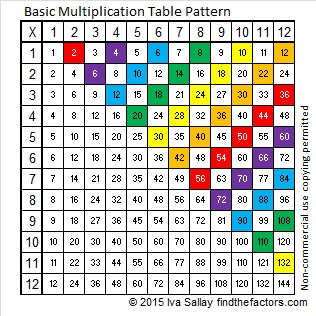If we look at the orange diagonal on the multiplication table above, we see 42, 40, 36, 30, 22, and 12. The differences between each consecutive member in that set are 2, 4, 6, 8, and 10. No matter which diagonal we choose the differences in the numbers follow that same pattern.

I asked myself, “Would there be a similar pattern if instead of multiplying two numbers together, we added their squares?” Let’s look at this table and see:On the orange diagonal we see 85, 89, 97, 109, 125, and 145. The differences between each consecutive member in this set are 4,  8, 12, 16, and 20. No matter which diagonal we choose the differences in the numbers will follow that same pattern.

Both of these tables can be extended infinitely in two directions and the patterns will hold true even for numbers on a diagonal that aren’t visible on a 12 x 12 table. For example, here are the numbers for the tables for such a diagonal.There is even an odd and rather square relationship between the product a x b and the sum a² + b²:

• (306 x 2) + 1 = 613
• (304 x 2) + 9 = 617
• (300 x 2) + 25 = 625
• (294 x 2) + 49 = 637
• (286 x 2) + 81 = 653
• (276 x 2) + 121 = 673 and so forth

So I ask again, Do you see a pattern? Mathematics is filled with them!

—————————————————————————————————

617 is the sum of the five prime numbers from 109 to 137

As mentioned previously 617 is the sum of two square numbers, specifically 19² + 16².

617 is the hypotenuse of the primitive Pythagorean triple 105-608-617 which was calculated using 19² – 16², 2(19)(16), and 19² + 16².

I learned from OEIS.org that 1!² + 2!² + 3!² + 4!² = 617.

—————————————————————————————————

• 617 is a prime number.
• Prime factorization: 617 is prime.
• The exponent of prime number 617 is 1. Adding 1 to that exponent we get (1 + 1) = 2. Therefore 617 has exactly 2 factors.
• Factors of 617: 1, 617
• Factor pairs: 617 = 1 x 617
• 617 has no square factors that allow its square root to be simplified. √617 ≈ 24.83948.How do we know that 617 is a prime number? If 617 were not a prime number, then it would be divisible by at least one prime number less than or equal to √617 ≈ 24.8. Since 617 cannot be divided evenly by 2, 3, 5, 7, 11, 13, 17, 19, or 23, we know that 617 is a prime number.

Here’s another way we know that 617 is a prime number: Since 19² + 16² = 617, an odd number, and 19 and 16 have no common prime factors, we know that 617 will be a prime number unless it is divisible by 5, 13, or 17. We can tell just by looking at it that it isn’t divisible by 5 or 17, but we will have to do the division to see if it is divisible by 13. Since 617 ÷ 13 = 47 R6, it cannot be evenly divided by 13, and thus we know that 617 is a prime number.

# 607 and Level 6

607 is the sum of the three prime numbers from 197 to 211.Print the puzzles or type the solution on this excel file: 10 Factors 2015-09-01

—————————————————————————————————

• 607 is a prime number.
• Prime factorization: 607 is prime.
• The exponent of prime number 607 is 1. Adding 1 to that exponent we get (1 + 1) = 2. Therefore 607 has exactly 2 factors.
• Factors of 607: 1, 607
• Factor pairs: 607 = 1 x 607
• 607 has no square factors that allow its square root to be simplified. √607 ≈ 24.637How do we know that 607 is a prime number? If 607 were not a prime number, then it would be divisible by at least one prime number less than or equal to √607 ≈ 24.6. Since 607 cannot be divided evenly by 2, 3, 5, 7, 11, 13, 17, 19, or 23, we know that 607 is a prime number.

—————————————————————————————————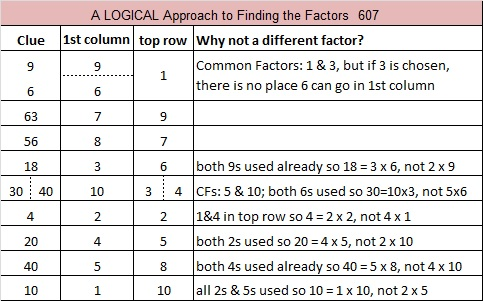# What often happens to a number like 599 when a number next to it has so many factors?

The square root of any number from 576 to 624 is between 24 and 25. That means the first number in any of their factor pairs will be 24 or less. The combined number of factor pairs for the 49 integers from 576 to 624 is 189. The number of factor pairs for any given number ranges from 1 to 12. Let’s look at the averages: The mean (189/49) is 3.857 factor pairs per number. The median is 3 factor pairs, and the mode (the number of factor pairs that occurs most often) is 4 factor pairs.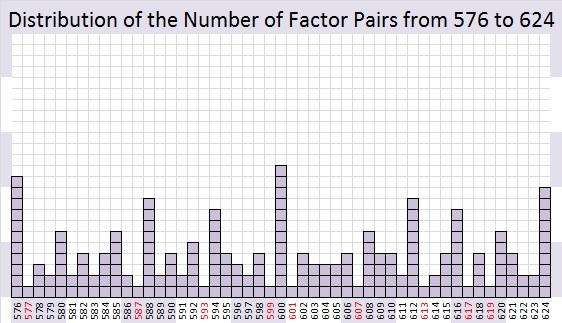What happens to a number like 599 when a number next to it has far more than the average number of factor pairs? Quite often, but not always, that number has no choice but to be a prime number.Even though 600 has only three prime factors (2, 3 and 5), it still managed to be divisible by 50% of the numbers from 1 to 24, and there just aren’t many possibilities left for the numbers immediately before or after it.

The twelve numbers less than or equal to 24 that will divide into 600 are 1, 2, 3, 4, 5, 6, 8, 10, 12, 15, 20 and 24.

Because 9, 14, 16, 18, 21, and 22 each have 2 or 3 as a prime factor, those six numbers also are not possible factors of 599 or 601.

Every whole number is divisible by 1, but besides that, there are only six numbers available as possible factors for those two numbers: 7, 11, 13, 17, 19, and 23. Since neither 599 nor 601 is divisible by any of those numbers, they turn out to be twin primes.

Usually at least one of the numbers before or after a number with far more than its fair share of factor pairs will be a prime number.

119 and 121, the numbers before and after 120 are notable exceptions. √120 ≈ 10.95441. The factors of 120 that are less than or equal to 10 are 1, 2, 3, 4, 5, 6, 8, and 10 which is 80% of the possible factors. Yet 119 managed to be divisible by 7, and 121 managed to be divisible by 11 so neither one of them is a prime number.

————————————————————————————-

If you add up the digits of 499, 589, or 598, you will get 22.

599 is the smallest whole number whose digits add up to 23. Thank you, OEIS.org for that number fact.

• 599 is a prime number and a twin prime with 601.
• Prime factorization: 599 is prime.
• The exponent of prime number 599 is 1. Adding 1 to that exponent we get (1 + 1) = 2. Therefore 599 has exactly 2 factors.
• Factors of 599: 1, 599
• Factor pairs: 599 = 1 x 599
• 599 has no square factors that allow its square root to be simplified. √599 ≈ 24.4744765How do we know that 599 is a prime number? If 599 were not a prime number, then it would be divisible by at least one prime number less than or equal to √599 ≈ 24.5. Since 599 cannot be divided evenly by 2, 3, 5, 7, 11, 13, 17, 19, or 23, we know that 599 is a prime number.

# 577 and Level 6

24² + 1 = 577 means 577 is the hypotenuse of a primitive Pythagorean triple which happens to be 48-575-577.Print the puzzles or type the solution on this excel file: 10 Factors 2015-08-03

—————————————————————————————————

• 577 is a prime number.
• Prime factorization: 577 is prime.
• The exponent of prime number 577 is 1. Adding 1 to that exponent we get (1 + 1) = 2. Therefore 577 has exactly 2 factors.
• Factors of 577: 1, 577
• Factor pairs: 577 = 1 x 577
• 577 has no square factors that allow its square root to be simplified. √577 ≈ 24.020824How do we know that 577 is a prime number? If 577 were not a prime number, then it would be divisible by at least one prime number less than or equal to √577 ≈ 24.02. Since 577 cannot be divided evenly by 2, 3, 5, 7, 11, 13, 17, 19, or 23, we know that 577 is a prime number.

—————————————————————————————————# 571 Family Time

For the last two weeks we’ve been spending time with our grandchildren in Salt Lake City, Utah; Portland, Oregon; Houston, Texas; and Hartford, Connecticut. We also spent time with my brother’s daughter and her two children. AND we celebrated my sister’s 70th birthday party and reconnected with all of her children in Portland. We have been busy, and I am tired, but happy.

We’ve had a wonderful time with all of them, and it was so painful to say goodbye. We and a few other people took pictures except we didn’t get any pictures of the grandkids that live within 10 miles of our home. What’s with that? I’m as guilty as anyone else who takes people and things for granted.

Here’s a picture of me holding my newest grandson. My daughter is in the background holding one of her nieces.———————————————————————————–

Since 171 + 190 + 210 = 571, it is the sum of the 18th, 19th, and 20th triangular numbers. That makes 571 the 20th centered triangular number.

• 571 is a prime number and a twin prime with 569.
• Prime factorization: 571 is prime.
• The exponent of prime number 571 is 1. Adding 1 to that exponent we get (1 + 1) = 2. Therefore 571 has exactly 2 factors.
• Factors of 571: 1, 571
• Factor pairs: 571 = 1 x 571
• 571 has no square factors that allow its square root to be simplified. √571 ≈ 23.8956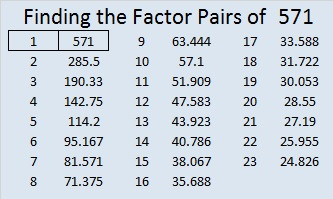How do we know that 571 is a prime number? If 571 were not a prime number, then it would be divisible by at least one prime number less than or equal to √571 ≈ 23.9. Since 571 cannot be divided evenly by 2, 3, 5, 7, 11, 13, 17, 19, or 23, we know that 571 is a prime number.

# 569 and Level 5

When we divide the last two digits of prime number 569 by 4, we get a remainder of one. That means that 569 is the sum of two square numbers, specifically, 20² + 13² = 569.

From those two square numbers we will obtain 569 as the hypotenuse of the primitive Pythagorean triple 231-520-569:

• 20² – 13² = 231
• 2 x 13 x 20 = 520
• 20² + 13² = 569Print the puzzles or type the solution on this excel file: 12 Factors 2015-07-27

—————————————————————————————————

• 569 is a prime number.
• Prime factorization: 569 is prime.
• The exponent of prime number 569 is 1. Adding 1 to that exponent we get (1 + 1) = 2. Therefore 569 has exactly 2 factors.
• Factors of 569: 1, 569
• Factor pairs: 569 = 1 x 569
• 569 has no square factors that allow its square root to be simplified. √569 ≈ 23.85372How do we know that 569 is a prime number? If 569 were not a prime number, then it would be divisible by at least one prime number less than or equal to √569 ≈ 23.8. Since 569 cannot be divided evenly by 2, 3, 5, 7, 11, 13, 17, 19, or 23, we know that 569 is a prime number.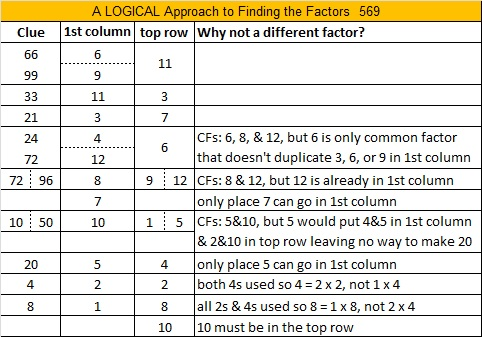# 564 and Level 6

Consecutive primes 281 and 283 add up to 564.

564 is made from three consecutive numbers so it can be evenly divided by 3. If the middle number is divisible by 3, then a number made from three consecutive numbers will also be divisible by 9. Is 564 divisible by 9? Why or why not?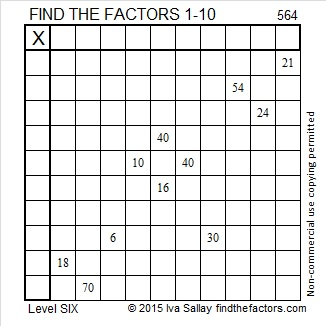Print the puzzles or type the solution on this excel file: 10 Factors 2015-07-20

—————————————————————————————————

• 564 is a composite number.
• Prime factorization: 564 = 2 x 2 x 3 x 47, which can be written 564 = (2^2) x 3 x 47
• The exponents in the prime factorization are 2, 1, and 1. Adding one to each and multiplying we get (2 + 1)(1 + 1)(1 + 1) = 3 x 2 x 2 = 12. Therefore 564 has exactly 12 factors.
• Factors of 564: 1, 2, 3, 4, 6, 12, 47, 94, 141, 188, 282, 564
• Factor pairs: 564 = 1 x 564, 2 x 282, 3 x 188, 4 x 141, 6 x 94, or 12 x 47
• Taking the factor pair with the largest square number factor, we get √564 = (√4)(√141) = 2√141 ≈ 23.74868—————————————————————————————————